# Difference between revisions of "Linear Systems"

 Prev: Dynamic Behavior Chapter 5 - Linear Systems Next: State Feedback

Previous chapters have focused on the dynamics of a system with relatively little attention to the inputs and outputs. This chapter gives an introduction to input/output behavior for linear systems and shows how a nonlinear system can be approximated near an equilibrium point by a linear model.

## Textbook Contents

• 1. Introduction
• 2. Properties of Linear Systems
• 3. Stability and Performance
• 4. Second Order Systems
• 5. Linearization
• 7. Exercises

## Chapter Summary

This chapter introduces the analysis tools for linear input/output systems.

1. A linear system is one in which the output is jointly linear in the intitial condition for the system and the input to the system. In particular, a linear system has the property that if we apply an inputwith zero initial condition, the corresponding output will be, whereis the output associated with the input. This propery is called linear superposition.

2. A differential equation of the formis a single-input, single-output (SISO) linear differential equation. Its solution can be written in terms of the matrix exponential

The solution to the differential equation is given by the convolution equation3. A linear systemis asymptotically stable if and only if all eigenvalues ofall have strictly negative real part and is unstable if any eigenvalue ofhas strictly positive real part. For systems with eigenvalues having zero real-part, stability is determined by using the Jordan normal form associated with the matrix. A system with eigenvalues that have no strickly positive real part is stable if and only if the Jordan block corresponding to each eigenvalue with zero part is a scalar (1x1) block.

4. The input/output response of a (stable) linear system contains a transient region portion, which eventually decays to zero, and a steady state portion, which persists over time. Two special responses are the step response, which is the output corresponding to an step input applied atand the frequency response, which is the response of the system to a sinusoidal input at a given frequency.

5. The step response is characterized by the following parameters:

• The steady state value,, of a step response is the final level of the output, assuming it converges.
• The rise time,, is the amount of time required for the signal to go from 10% of its final value to 90% of its final value.
• The overshoot,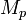, is the percentage of the infal value by which the signal initially rises above the final value.
• The settling time,, is the amount of time required for the signal to stay within 5% of its final value for all future times.

6. The frequency response is given by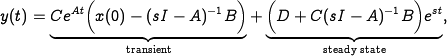whereand. The gain and phase of the frequency response are given by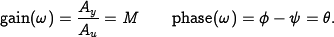7. A nonlinear system of the formis a single-input, single-output (SISO) nonlinear system. It can be linearized about an equibrium point,,by defining new variables

The dynamics of the system near the equilibrium point can then be approximated by the linear system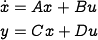where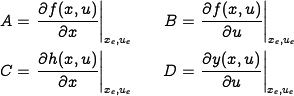The equilibrium point for a nonlinear system is locally asymptotically stable if the real part of the eigenvalues of the linearization about that equilibrium point have strictly negative real part.